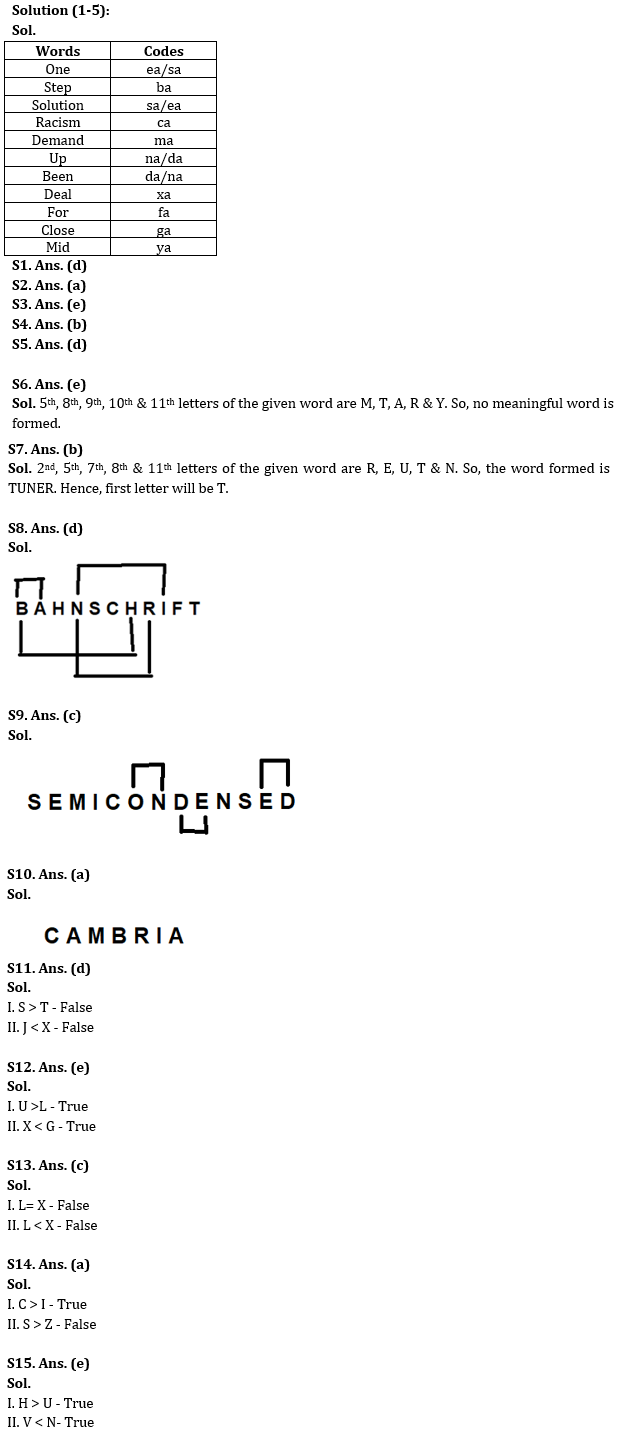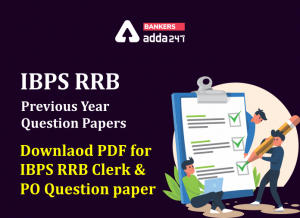Latest Banking jobs   »

# Reasoning Ability Quiz For IBPS RRB PO Prelims 2022- 28th June

Directions (1-5): Read the following information carefully to give the answers to the questions.
In a certain code language,
‘One step solution racism’ is coded as ‘ba sa ea ca’
‘Racism demand deal for’ is coded as ‘xa fa ma ca’
‘Close demand up been’ is coded as ‘na da ma ga’
‘Mid close for step’ is coded as ‘ga fa ba ya’

Q1. What is the code of ‘demand’?
(a) xa
(b) ga
(c) da
(d) ma
(e) None of the above

Q2. Which is the following word is coded as ‘ya’?
(a) Mid
(b) Been
(c) One
(d) Race
(e) None of the above

Q3. What is the code of ‘racism solution’?
(a) ca ea
(b) da na
(c) ca sa
(d) ma xa
(e) Either (a) or (c)

Q4. Which is the following word is coded as ‘fa’?
(a) One
(b) For
(c) Demand
(d) Deal
(e) None of the above

Q5. What is the code of ‘deal’?
(a) ya
(b) da
(c) na
(d) xa
(e) None of the above

Q6. If it is possible to make a five-letter meaningful word from 5th, 8th, 9th, 10th & 11th letters from the left end of the of word “DOCUMENTARY”, then which will be the 3rd letter of that meaningful word? If no meaningful word is formed, then mark the answer as X. If more than one meaningful word is formed then, mark the answer as Z.
(a) A
(b) T
(c) Z
(d) R
(e) X

Q7. If it is possible to make a five-letter meaningful word from 2nd, 5th, 7th, 8th & 11th letters from the left end of the of word “PROSECUTION”, then which will be the first letter of that meaningful word? If no meaningful word is formed, then mark the answer as X. If more than one meaningful word is formed then, mark the answer as Z.
(a) N
(b) T
(c) U
(d) Z
(e) X

Q8. How many pairs of letters are there in the word “BAHNSCHRIFT” which has as many letters between them as in the alphabetical series (both forward and backward direction)?
(a) One
(b) Two
(c) Three
(d) Four
(e) None of the above

Q9. How many pairs of letters are there in the word “SEMICONDENSED” which has as many letters between them as in the alphabetical series (both forward and backward direction)?
(a) One
(b) Two
(c) Three
(d) Four
(e) None of the above

Q10. How many pairs of letters are there in the word “CAMBRIA” which has as many letters between them in the word as in the alphabetical series (both forward and backward direction)?
(a) None
(b) Two
(c) Three
(d) Four
(e) One

Directions (11-15): In these questions, relationship between different elements is shown in the statements. These statements are followed by two conclusions. Assuming the given statements are true, then find out which of the given conclusion(s) is/are definitely true.

Q11.
Statements:
S ≥ C <T = J> N > P = X> V
Conclusions:
I. S>T
II. J<X
(a) Only I is true
(b) Only II is true
(c) Either I or II is true
(d) Neither I nor II is true
(e) Both I and II are true

Q12. Statements:
G = U > B ≥ X < D = Z, B > L
Conclusions:
I. U>L
II. X < G
(a) Only I is true
(b) Only II is true
(c) Either I or II is true
(d) Neither I nor II is true
(e) Both I and II are true

Q13.
Statements:
X ≥ H > N = Z < Y ≤ S ≤ Q, H ≥ L
Conclusions:
I. L= X
II. L < X
(a) Only I is true
(b) Only II is true
(c) Either I or II is true
(d) Neither I nor II is true
(e) Both I and II are true

Q14. Statements:
S > N > L = I ≤ Z ≤ F = R < C
Conclusions:
I. C > I
II. S > Z
(a) Only I is true
(b) Only II is true
(c) Either I or II is true
(d) Neither I nor II is true
(e) Both I and II are true

Q15. Statements:
O ≤ T = H < N, O = V ≥ K > U, P ≤ N = D
Conclusions:
I. H>U
II. V <N
(a) Only I is true
(b) Only II is true
(c) Either I or II is true
(d) Neither I nor II is true
(e) Both I and II are true

Solutions#### Congratulations!Incorrect details? Fill the form again here

•Reasoning Ability Quiz For IBPS RRB PO C...
•Quantitative Aptitude Quiz For IBPS RRB ...
•Reasoning Ability Quiz For IBPS RRB PO C...
•Quantitative Aptitude Quiz For IBPS RRB ...
•IBPS RRB Previous Year Question Paper PD...
•Quantitative Aptitude Quiz For IBPS RRB ...